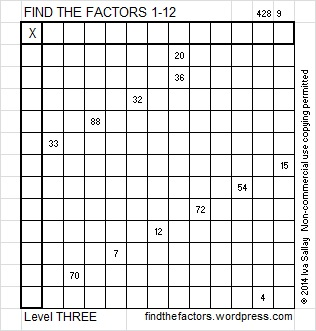# 176 and Level 3

 176 is a composite number. Factor pairs: 176 = 1 x 176, 2 x 88, 4 x 44, 8 x 22, or 11 x 16 Factors of 176: 1, 2, 4, 8, 11, 16, 22, 44, 88, 176 Prime factorization: 176 = 2 x 2 x 2 x 2 x 11 which can be written (2^4) x 11.Excel file of puzzles and previous week’s factor solutions: 12 Factors 2014-07-14

A Logical Approach to FIND THE FACTORS: Find the column or row with two clues and find their common factor. Write the corresponding factors in the factor column (1st column) and factor row (top row).  Because this is a level three puzzle, you have now written a factor at the top of the factor column. Continue to work from the top of the factor column to the bottom, finding factors and filling in the factor column and the factor row one cell at a time as you go.